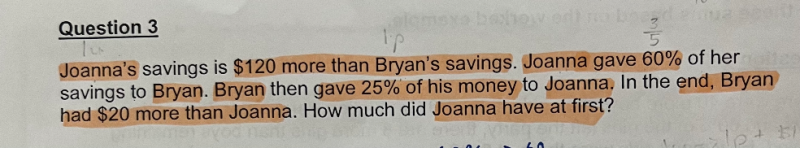# QuestionIf calculator is permitted for this question,  this method is straight forward in thinking using % without conversion to fractions or required to find the correct factor to use and all calculations can be done by using a calculator

Bryan                                                      Joanna

Initially                                                                       u                                                             u+ 120

60% of Joanna                                                                                                0.6(u+120) = 0.6u + 72

Transfer of J to B                        u +0.6u+72 = 1.6u + 72                           u+120-0.6u-72 =0.4u + 48

25% of Bryan                           0.25(1.6u + 72) = 0.4u +18

Transfer of B to J(Final)   1.6u+72-0.4u-18=  1.2u + 54                         0.4u+48+0.4u+18 =0.8u +66

Finally, Bryan has \$20 more than Joanna implies

1.2u + 54  – (0.8u +66) = 20

0.4u = 20+12 =32 => u = 80

Hence  at first, Joanna has (u+120)= 80+120 = \$200

0 Replies 1 Like

Let us assume Bryan have 10U initially,

Bryan                                      Joanna

Initially                                                 10U                                         10U+120

After J gave Bryan 60%         10U+6/10(10U+120)                           4/10(10U+120)=4U+48

=16U+72

After B gave J 25%               3/4(16U+72)                            1/4 (16U + 72) + 4U +48

=12U+54                                 =8U +66

So finally Bryan had \$20 more than Joanna,

12U + 54  = 8U +66 +20

4U = 32

U = 8

10*8 +120 = 200

So Joanna has \$200 dollars at first

0 Replies 1 Like
```Thinking:
- there is a transfer of 60% which is 3/5 (as you have indicated)
- there is a transfer of 25% which is 1/4
- there is division by 5 and 4... so let's try 20u

Working:
Joanna          Transfer           Bryan
=================================================
20u + 120                         20u
8u + 48   ==> 60%: 12u + 72 ==>  32u + 72
16u + 66   <== 25%:  8u + 18 <==  24u + 54

Bryan has (24u + 54) - (16u + 66) = 8u - 12 = 20 dollars
more than Joanna. That means 8u = 32 and u = 4.
So Joanna has 20u + 120 = \$200 at first.

Checking:
Joanna        Transfer         Bryan
=================================================
200                           80
80     ==> 60%:120 ==>      200
130     <== 25%: 50 <==      150
Bryan has 150-130 = 20 more than Joanna.```
0 Replies 1 Like